A venturimeter is a device used for measuring the rate of a flow of a fluid flowing through a pipe.
It consists of three parts.

1. A short converging part: It is that portion of the venturi where the fluid gets converges.
2. Throat: It is the portion that lies in between the converging and diverging part of the venturi. The cross section of the throat is much less than the cross section of the converging and diverging parts. As the fluid enters in the throat, its velocity increases and pressure decreases.
3. Diverging part: It is the portion of the venturimeter (venturi) where the fluid gets diverges

The working of venturimeter is based on the principle of Bernoulli’s equation.

Bernoulli’s Statement: it states that in a steady, ideal flow of an incompressible fluid, the total energy at any point of the fluid is constant. The total energy consists of pressure energy, kenetic energy and potential energy or datum energy.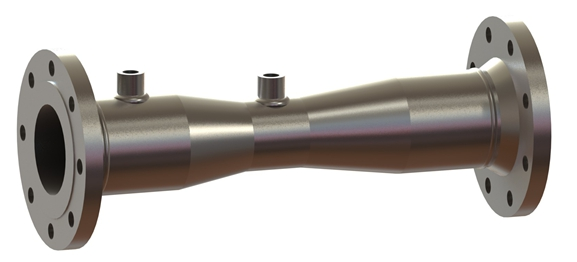## Practical to determine the coefficient of discharge for venturimeter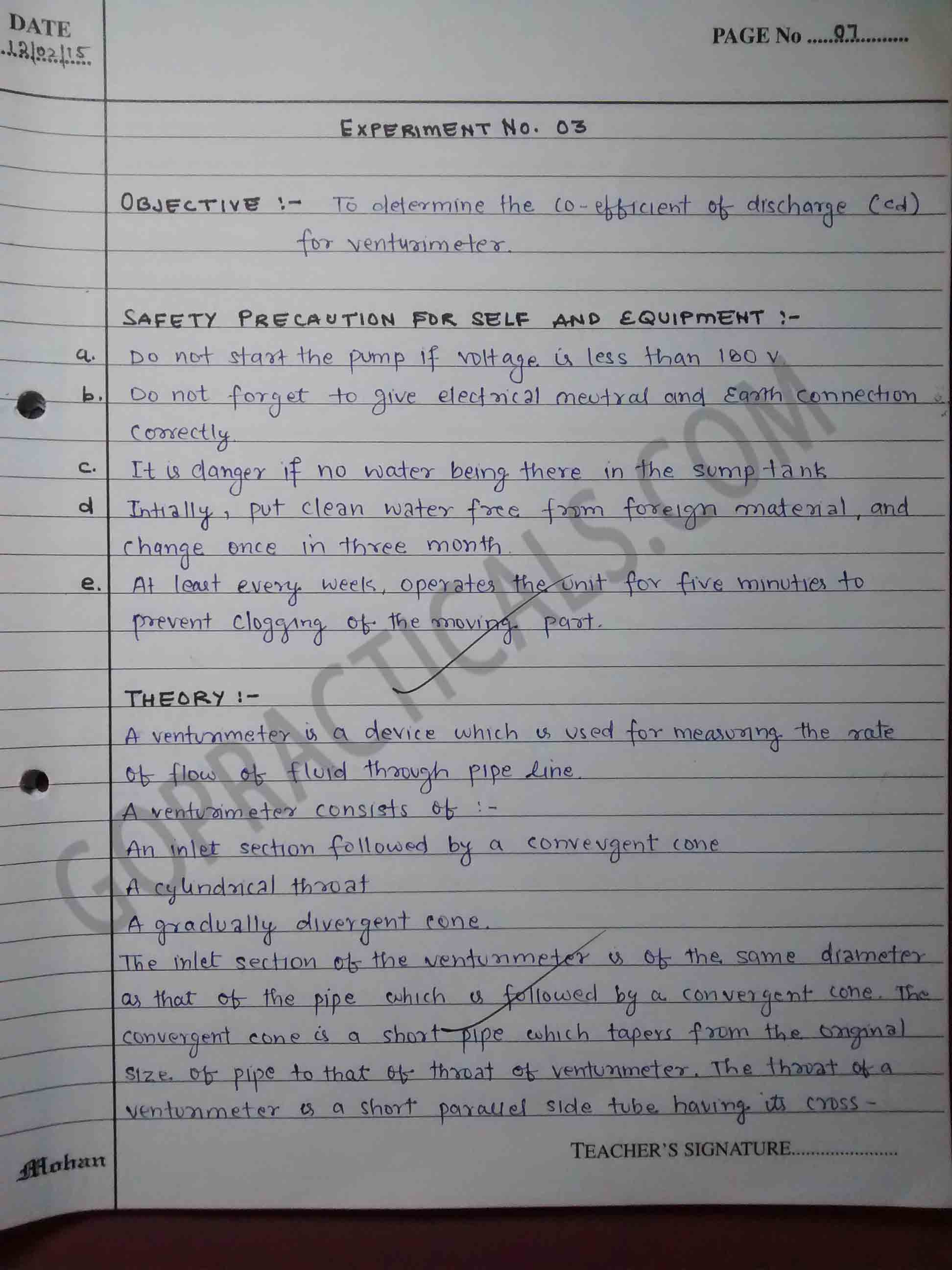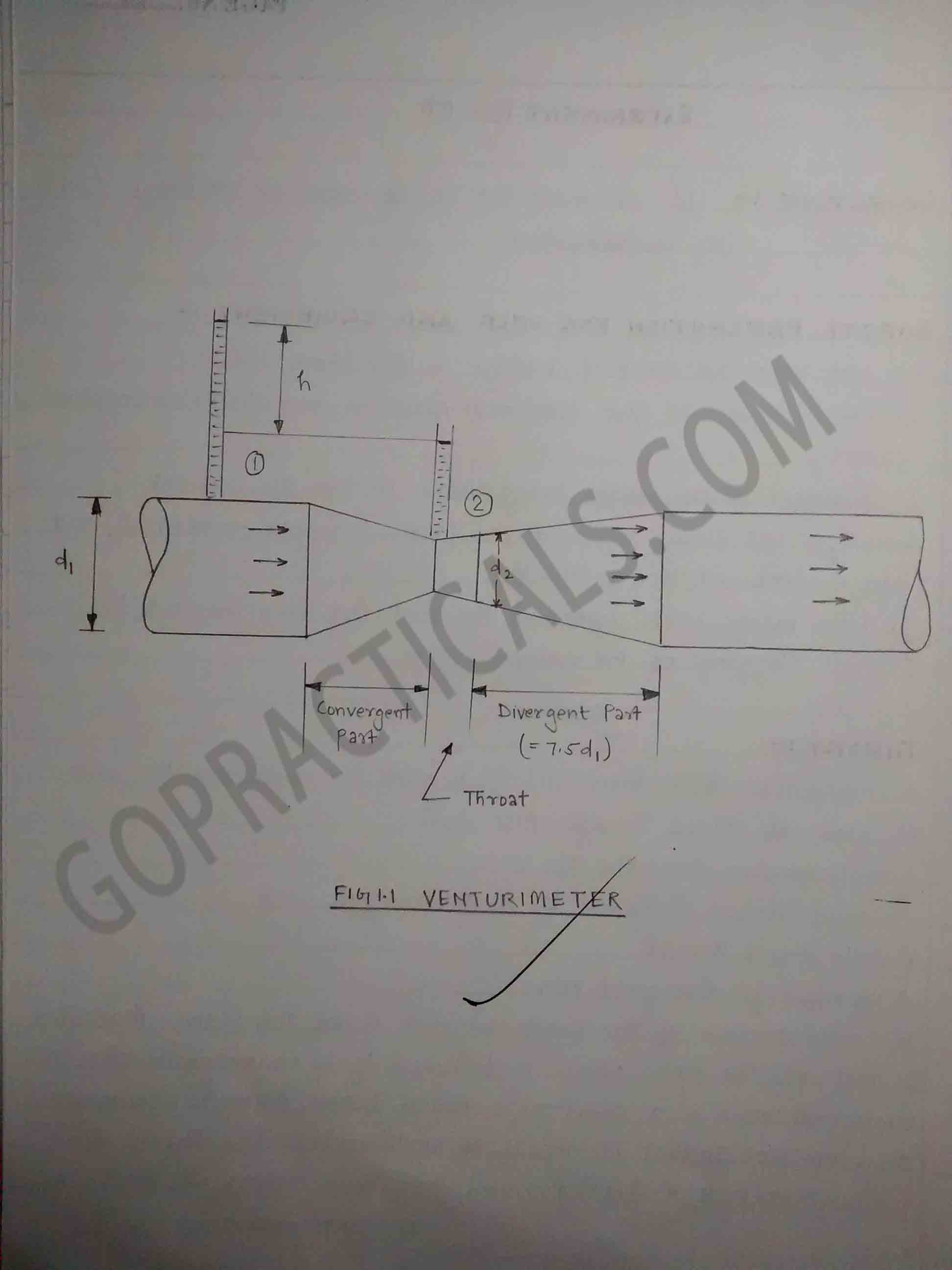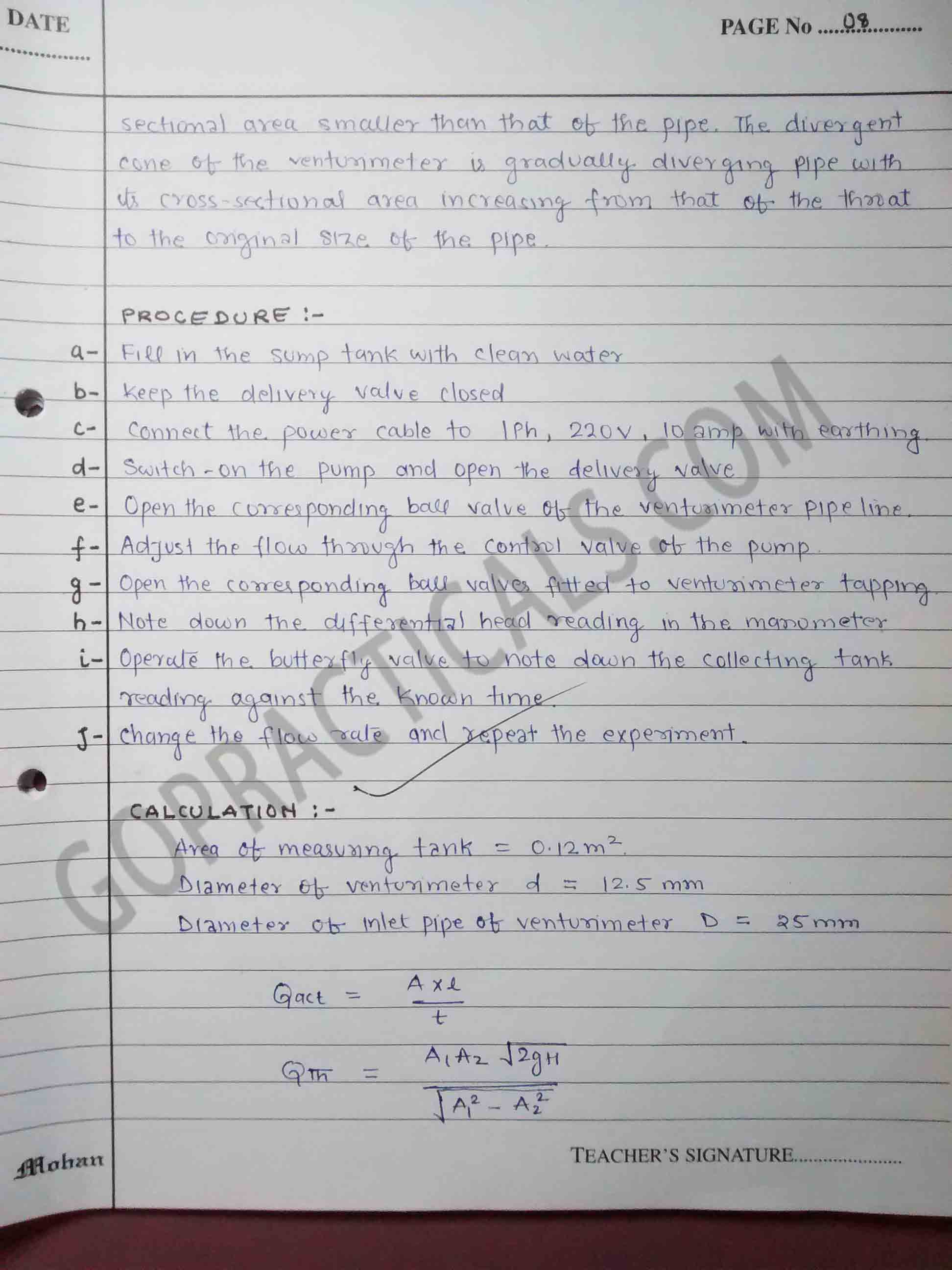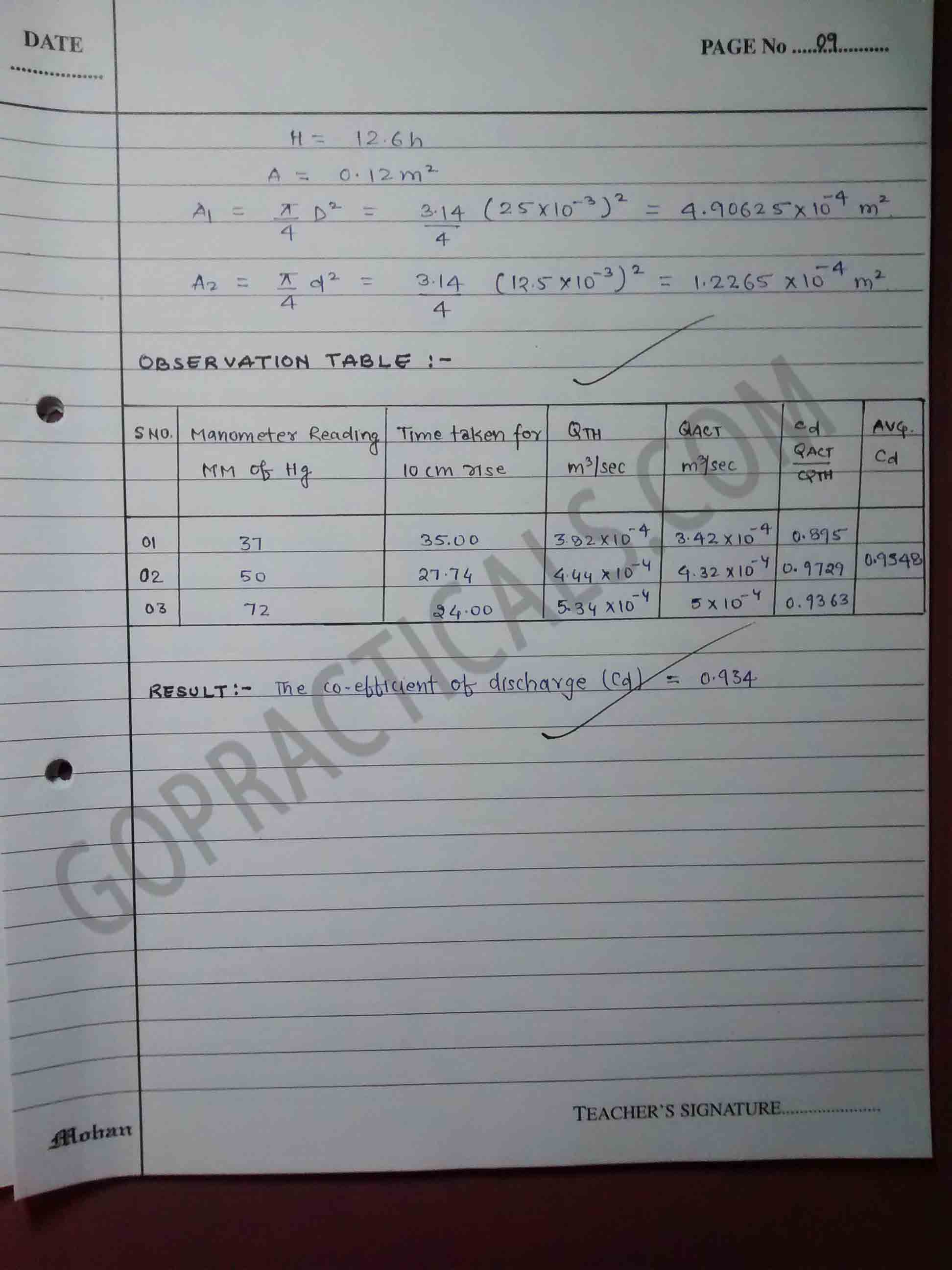## Watch this Video to know more about venturimeter

Also Check Out – Consistency of Concrete by Slump Cone

Thanks for visiting us…

Determination of Coefficient of discharge for Venturimeter – Fluid Practicals
Tagged on: